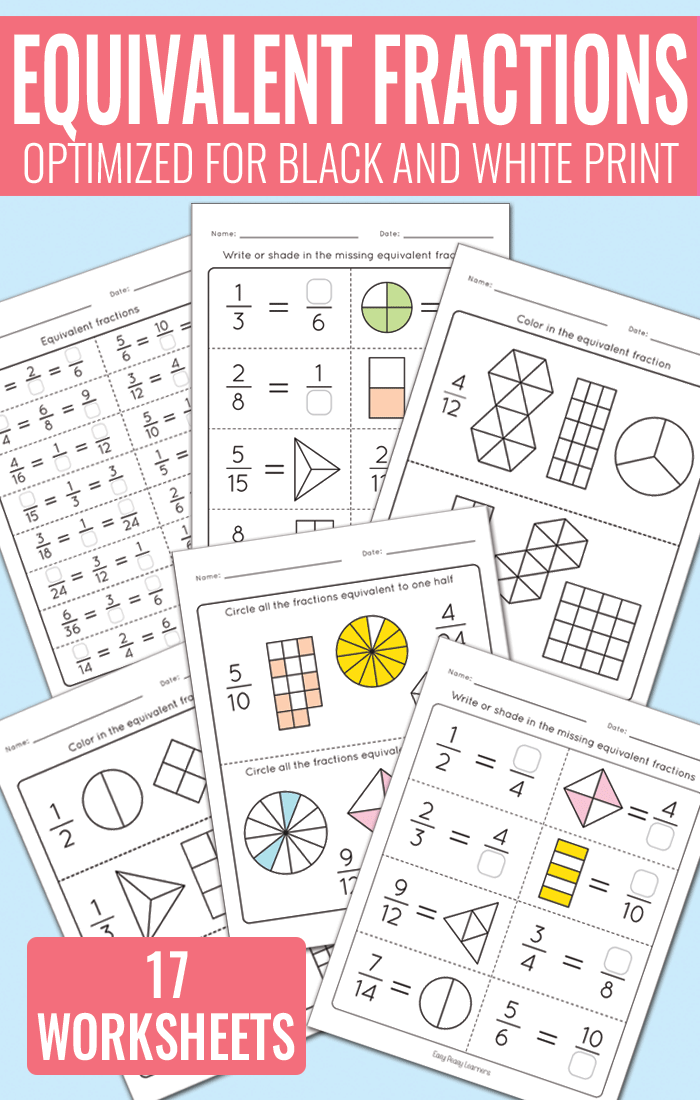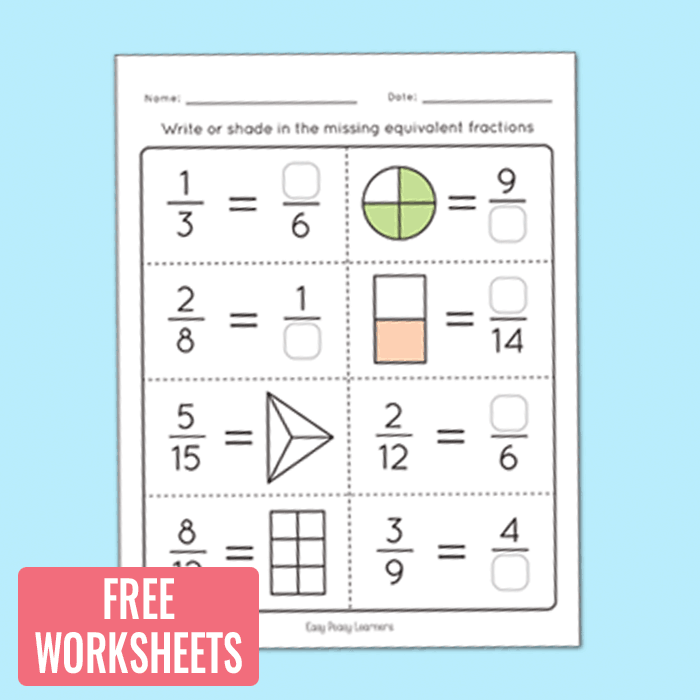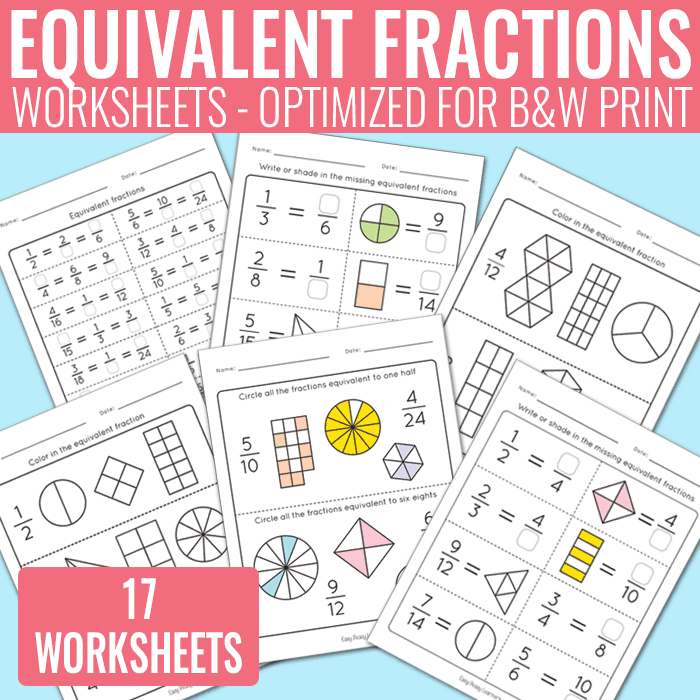# Equivalent Fractions Worksheets – Fractions Unit

Is two quarters the same as one half? Let your students practice equivalent fractions with this set of equivalent fractions worksheets.## Equivalent Fractions Worksheets

With 17 different worksheets your students will master equivalent fractions in no time

What you will get in this printable worksheet set;

• 17 worksheets + answer key
• 5 worksheets where the fraction is written and students are asked to color in the equivalent fractions in the given shapes
• 5 worksheets where studends are asked to write or shade in the missing equivalent fraction with either the numerator, denominator or shaded part of the shape is given.
• 2 worksheets where students find equivalent fractions by either finding the numerator or denominator.
• 5 worksheets where the fraction is written down with words and students are asked to circle all the equivalent fractions.

These are great for student homework or math test prep.

You can get the whole set at Teachers Pay Teachers where you can also preview the whole set.

Get Equivalent Fractions Math Worksheets

Get Equivalent Fractions Worksheets

Educators can laminate these worksheets to be used with dry erase markers or page protectors. This set comes in full color but will print nicely as black and white too.Want to try out these worksheets? Print this freebie page

Grab the equivalent fraction worksheet